## lp billiard

Here are some higher resolution phase space pictures of the lp billiard investigated first in 1996 with Monwhea (Momo) Jeng. The lp ball is the unit ball in the lp plane. The case p=infinity is the Taxi metric where the table like in the p=1 case is a square and so integrable. Of course also the Euclidean metric p=2 is integrable. We believe that the other cases have positive metric entropy. The cases p=2n are especially interesting as then the map is real analytic, the curves being algebraic curves then. Especially interesting is the simplest case x4 +y4 = 1. Unknown there is also (a questions I asked in this article) in which cases the return map is algebraic in some coordinates.
The cases p=1,2 and infinity are the only integrable cases because the topological entropy is positive for the other p. This combines theorems of Mather (flat points), Hubacher (singularities in curvature) and Angenent (non-existence of a foliation of caustic implies positive topological entropy). Nothing about the metric entropy (Kolmogorov-Sinai entropy) is known. We have measured it numerically in that paper and also seen numerically an interesting phase transition at p=2. The entropy h(p)/log|p-2| appears asymptotically linear near p=2 with different slopes on the left and right hand side.
The existence of presumably dense set of periodic orbits (associated each with possibly tiny elliptic stability islands) makes the entropy problem difficult. When I started to work on the problem of positive Lyapunov exponents in my undergraduate thesis, I tried transport analysis: look at the cocycle F(x,u) = (Tx,dT(x)) on the projective tangent bundle which is highly dissipative there as in the case of positive Lyapunov exponents, things attract on a Ledrapier curve (x,u(x)), where u(x) are the Oseledec directions. I still now, after 34 years think that this is the way to go but that one needs to develop some serious functional analytic estimation tools and first work in a case where the maps are real analytic like the l4 case. I have tried for years to push the Herman subharmonic and plurisubharmonic tools (Sorets, Spencer, Bourgain have made in that area serious progress) but subharmonic estimates seem not to work for billiards. An other idea I had been pursuing in my thesis is to note that the entropy of a discrete Hamiltonian system is log(det(L)) for some ``random Jacobi matrix". I use the name ``random" in the sense that they are operator valued random variables and not that there is any randomness assumed. In probability theory, a random variable is just a measurable function from the probability space to the reals. In quantum mechanics and solid state physics, one looks at random variables which are operator valued. The operator L is in some nice von Neumann algebra with trace and so has a nice determinant. This then in turn has relations with spectral theory of operators and I could learn quite a bit more about this at Caltech. But rewriting a number as a determinant does not help to compute the number yet. So, I had already in my thesis deformed the operators in an isospectral way. This involves an other exciting area of mathematics, the theory of integrable systems which in finite dimensions essentially boils down to algebraic geometry as the Toda flows are linearized by looking at the motion of the divisors on the Jacobean. The Abel-Jacobi map then linearlizes the system. The determinant of course remains the same under deformation and one could hope that one can compute the determinant possibly in a limit or average. This did not work neither but in my thesis, this allowed me to write about infinite dimensional Toda systems.
For a recent lecture in spring 2021 I made some high resolution phase space pictures for some parameters p=1.25, 1.5, 1.75, 2.125, 2.25,2.5,2.75,3,3.5,4,5,6,7,8,9 and 12. Color encodes Lyapunov exponent. The red orbits have zero Lyapunov exponent (typically KAM tori), the blue orbits appear to be chaotic. Click to see larger pictures.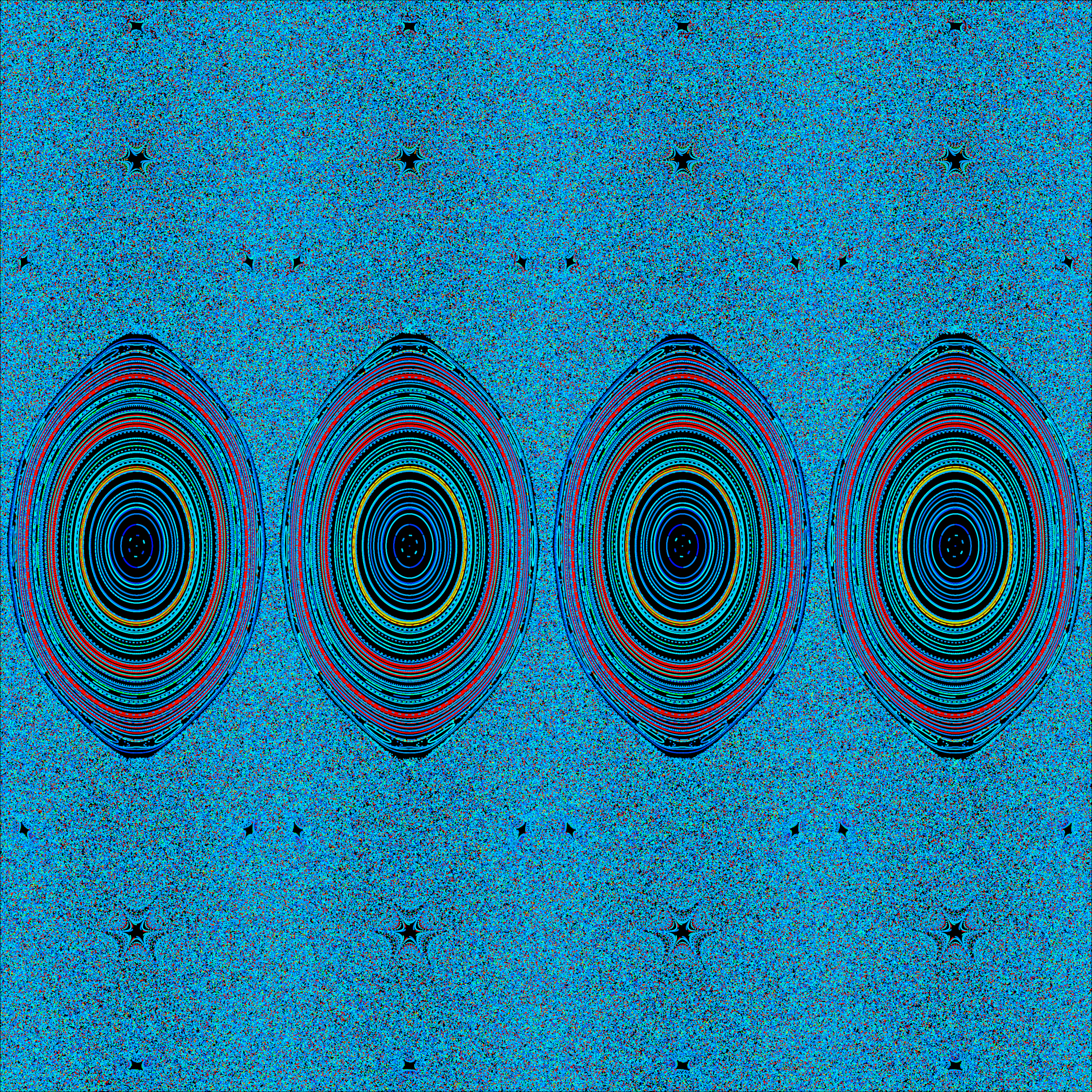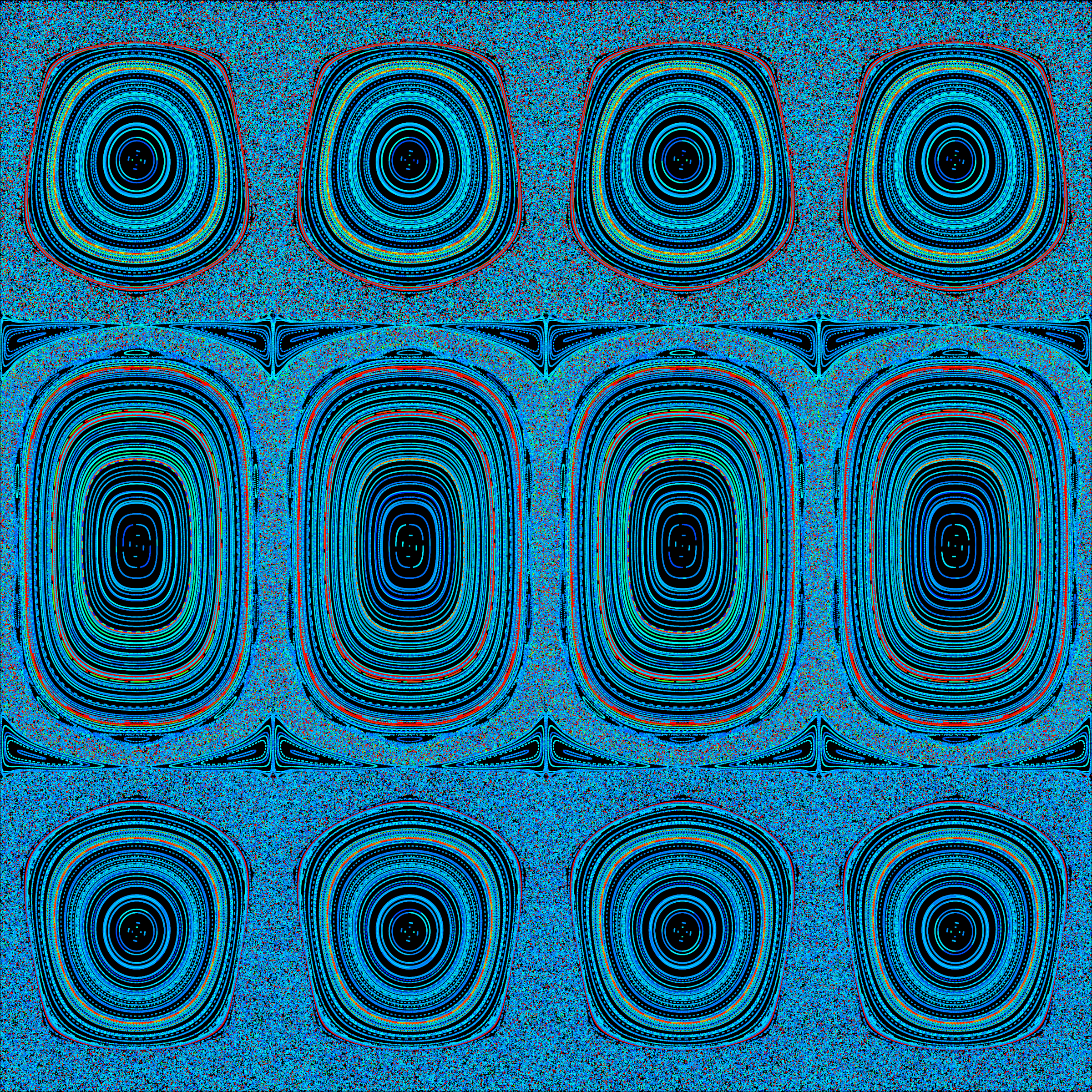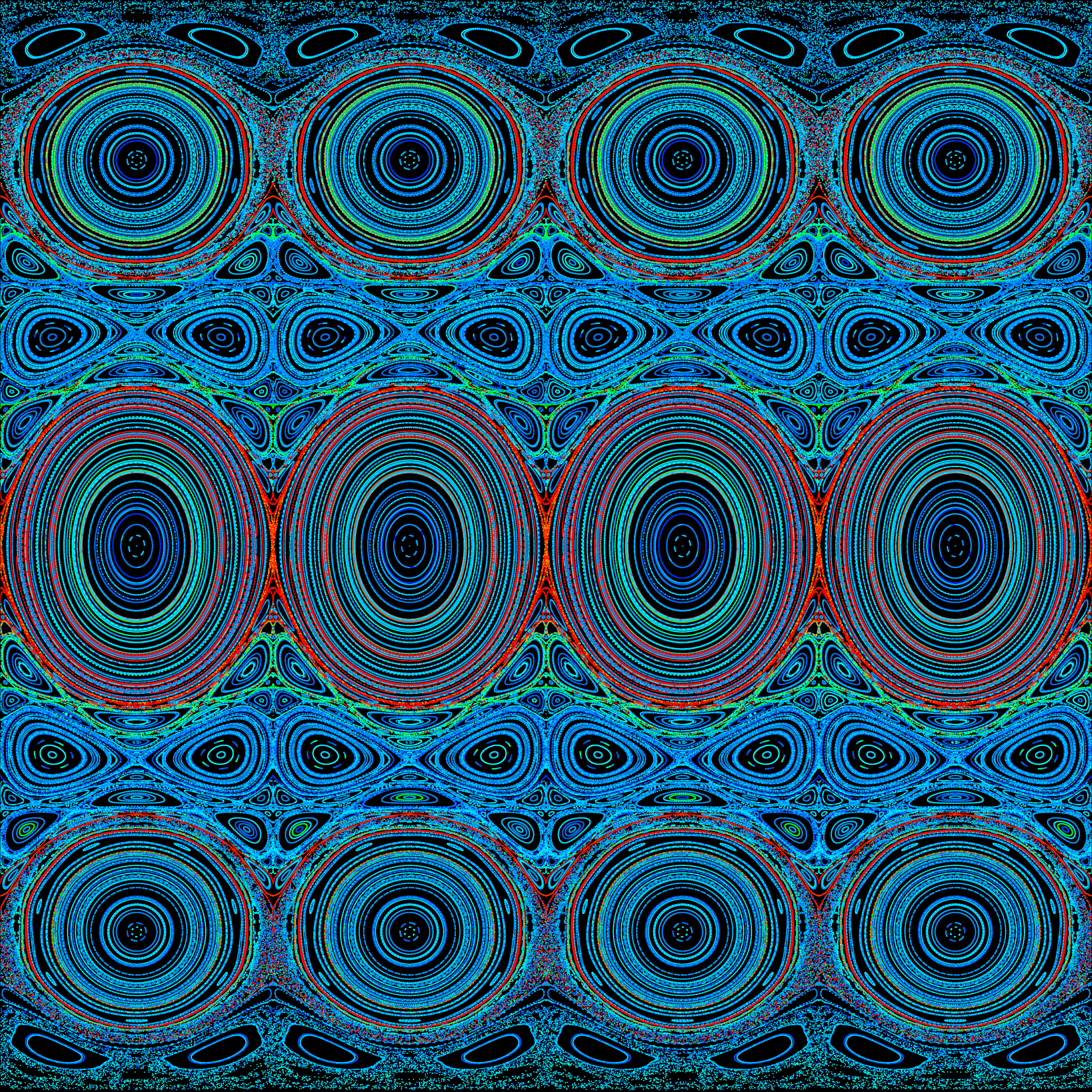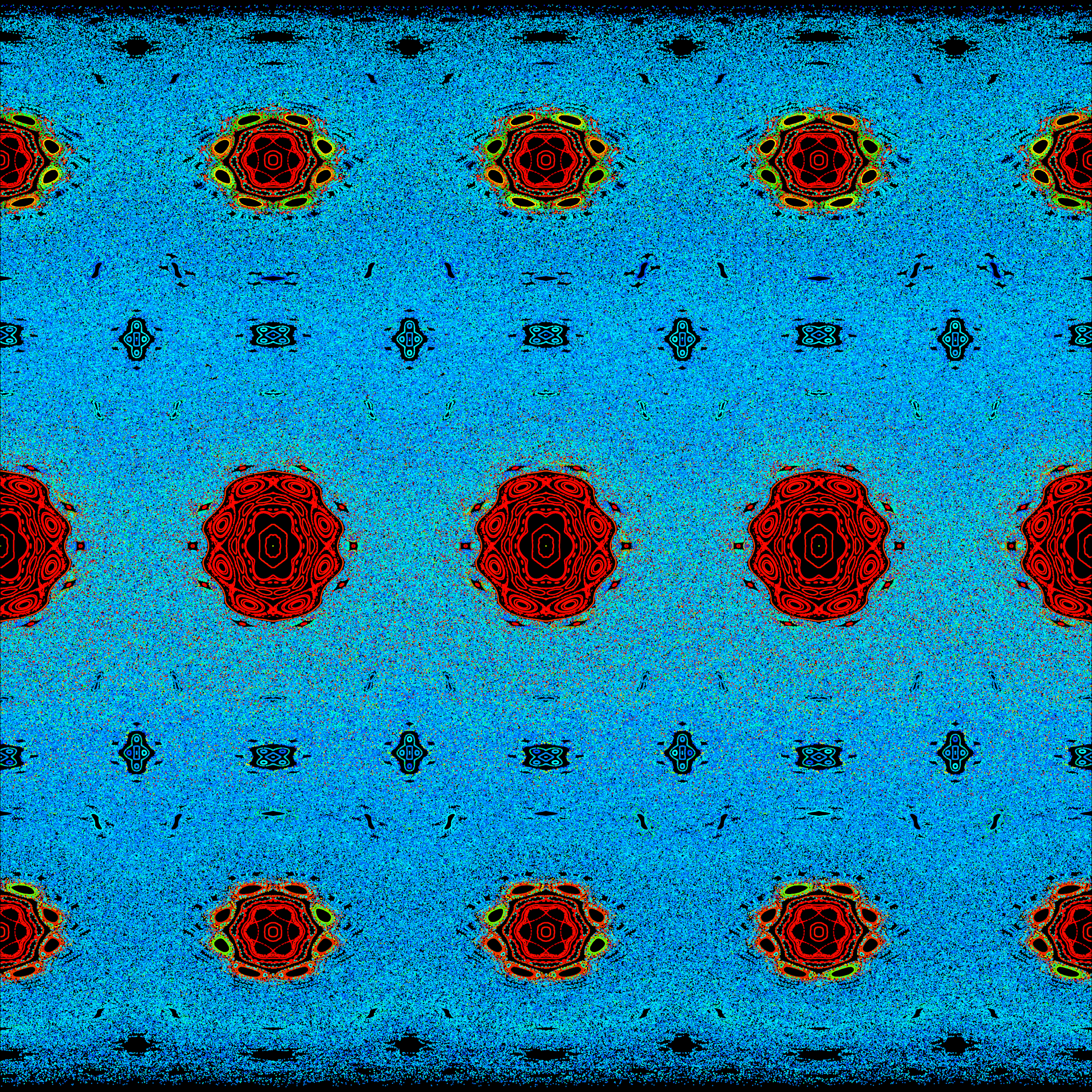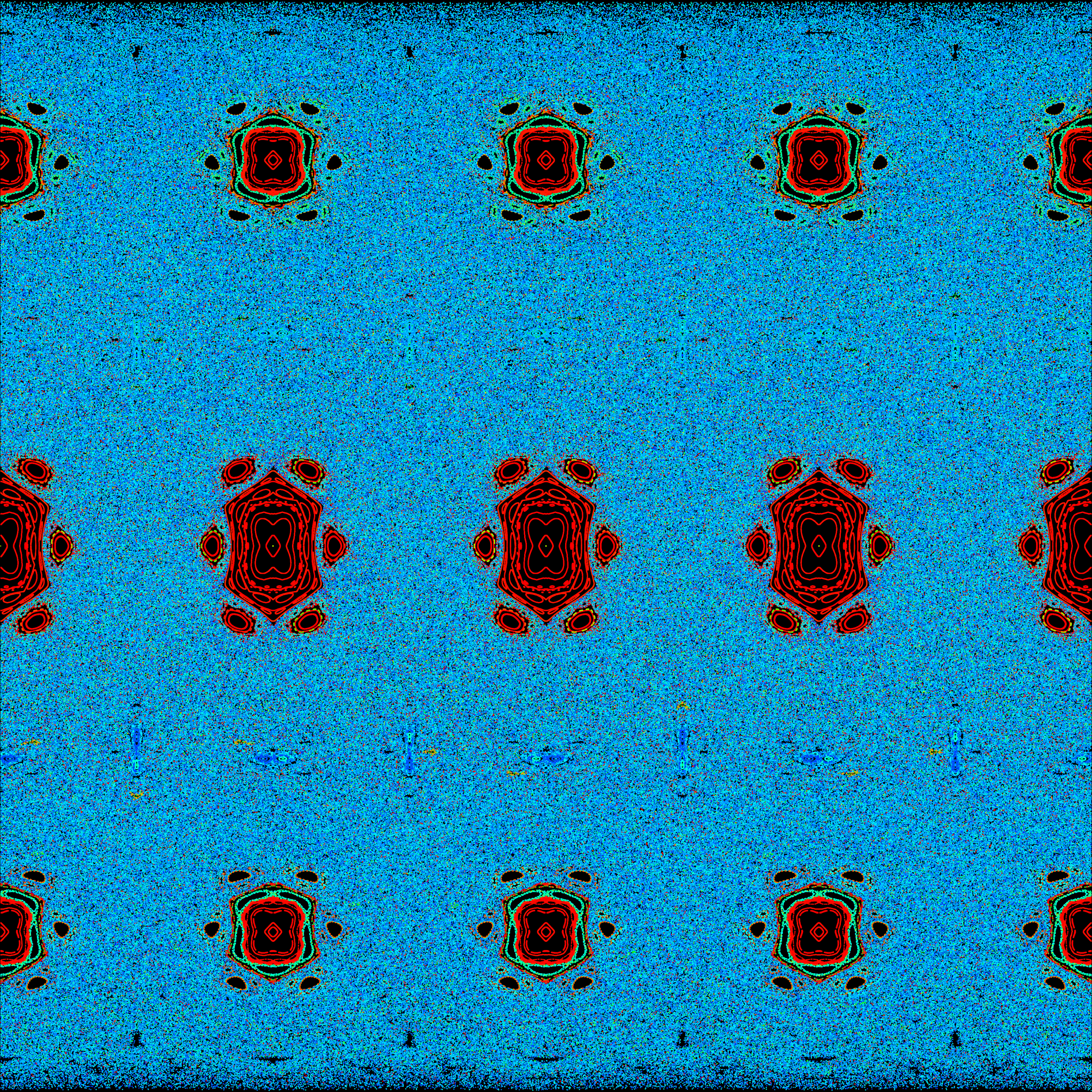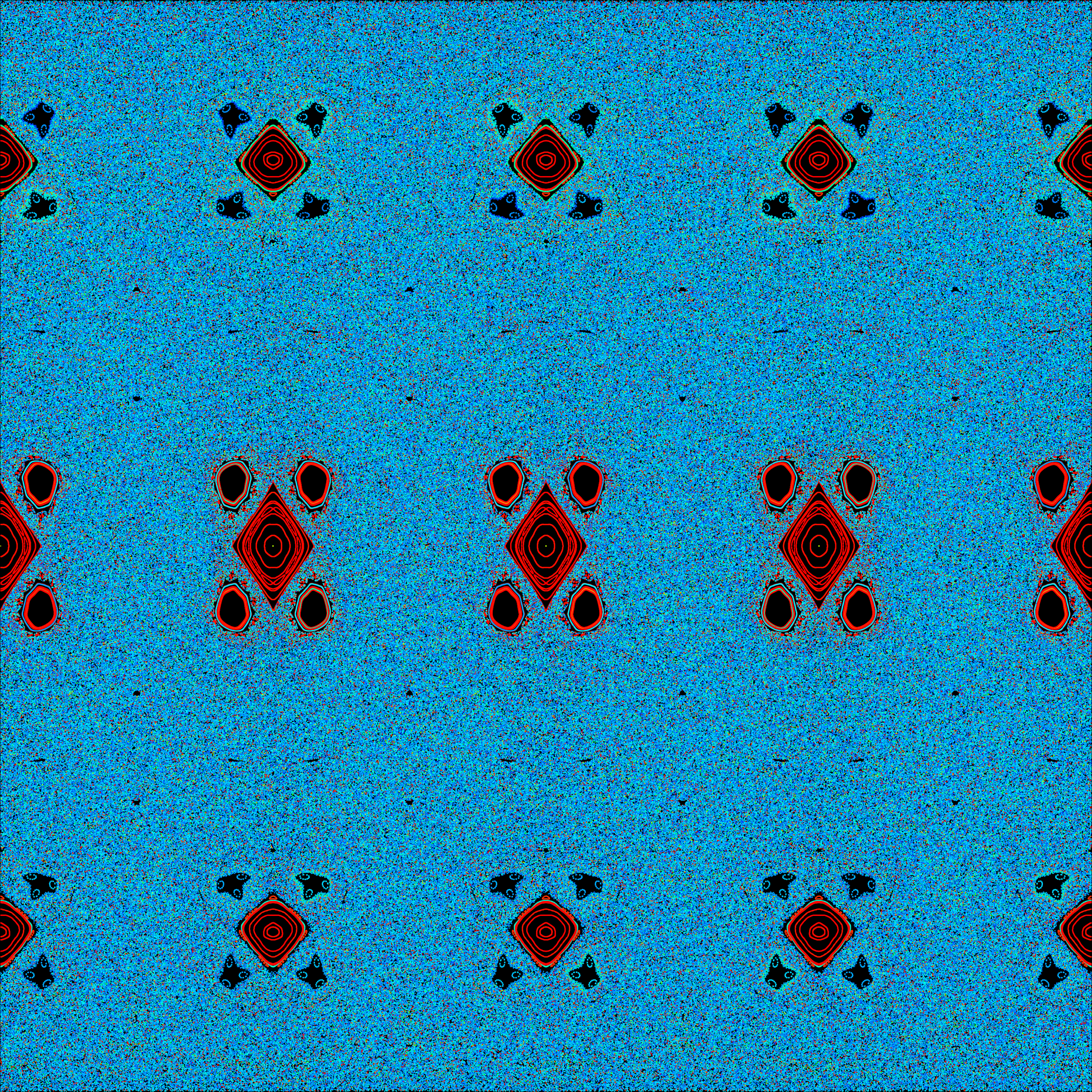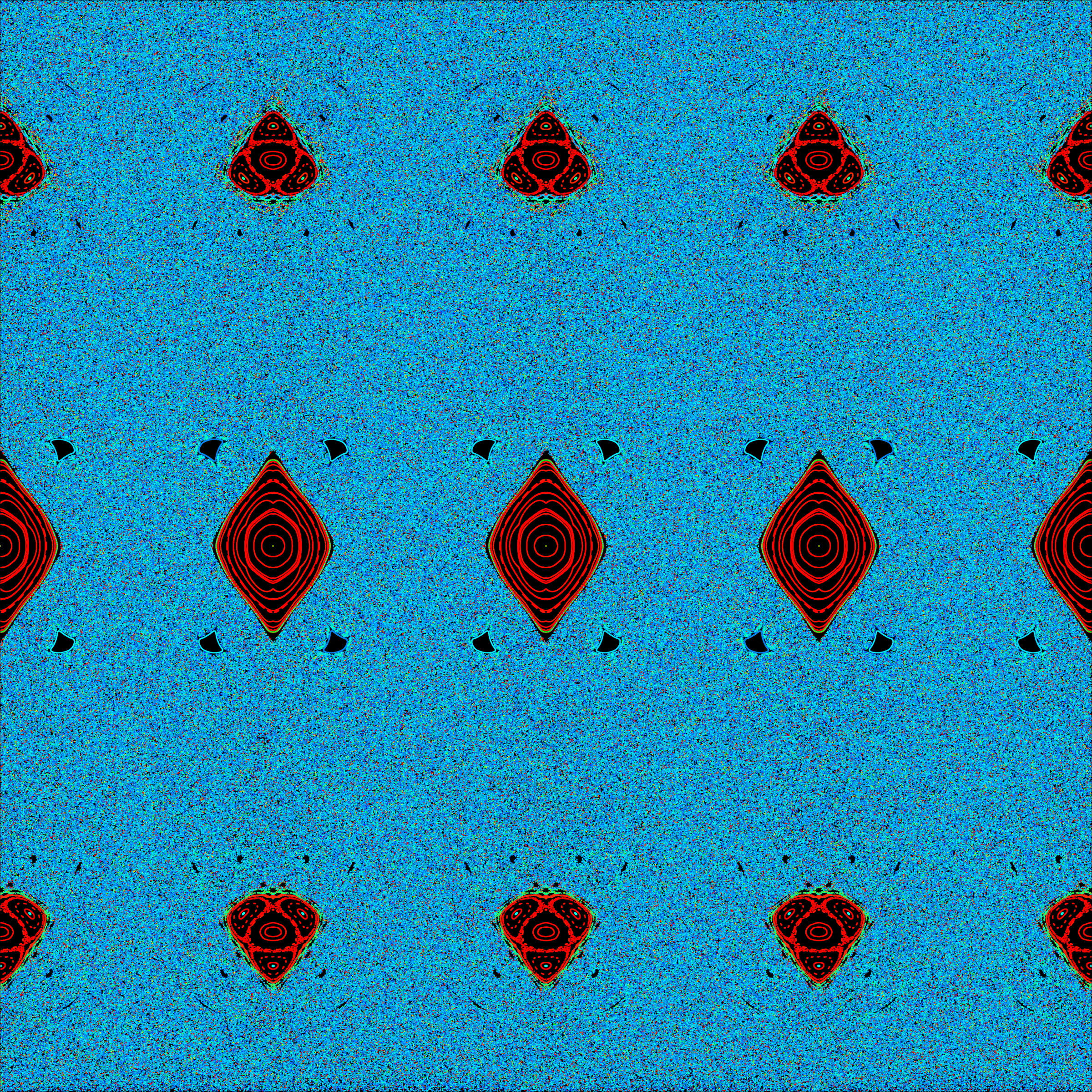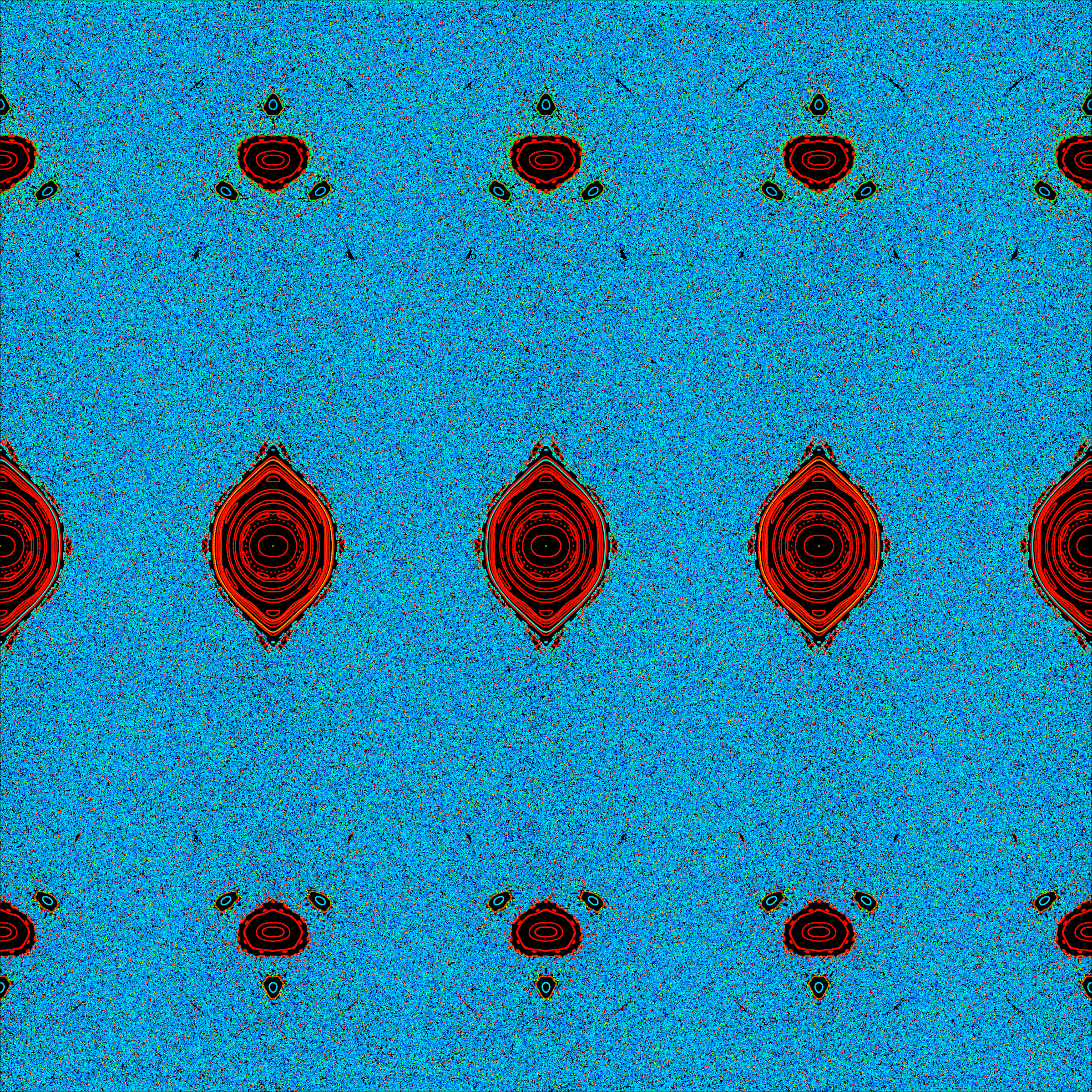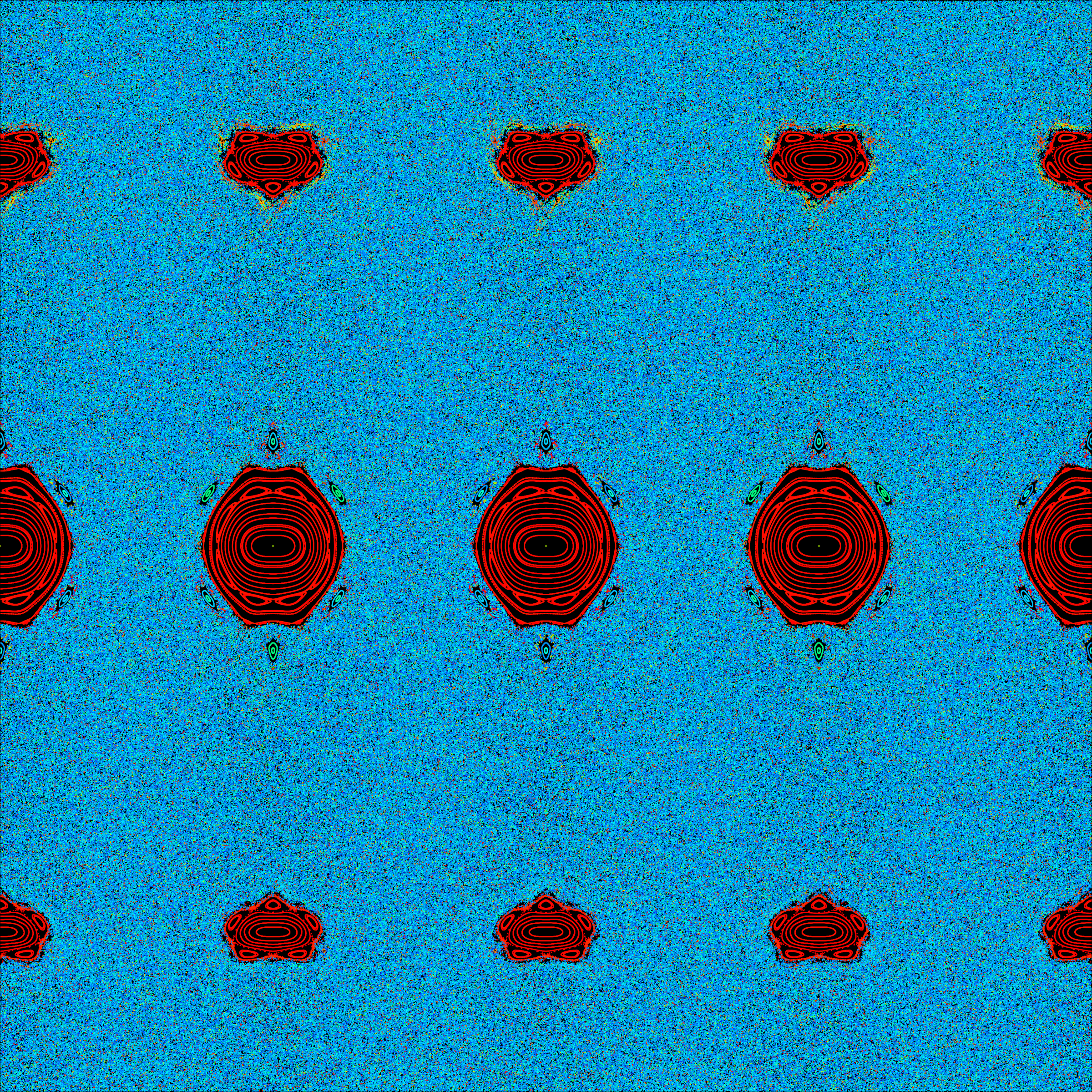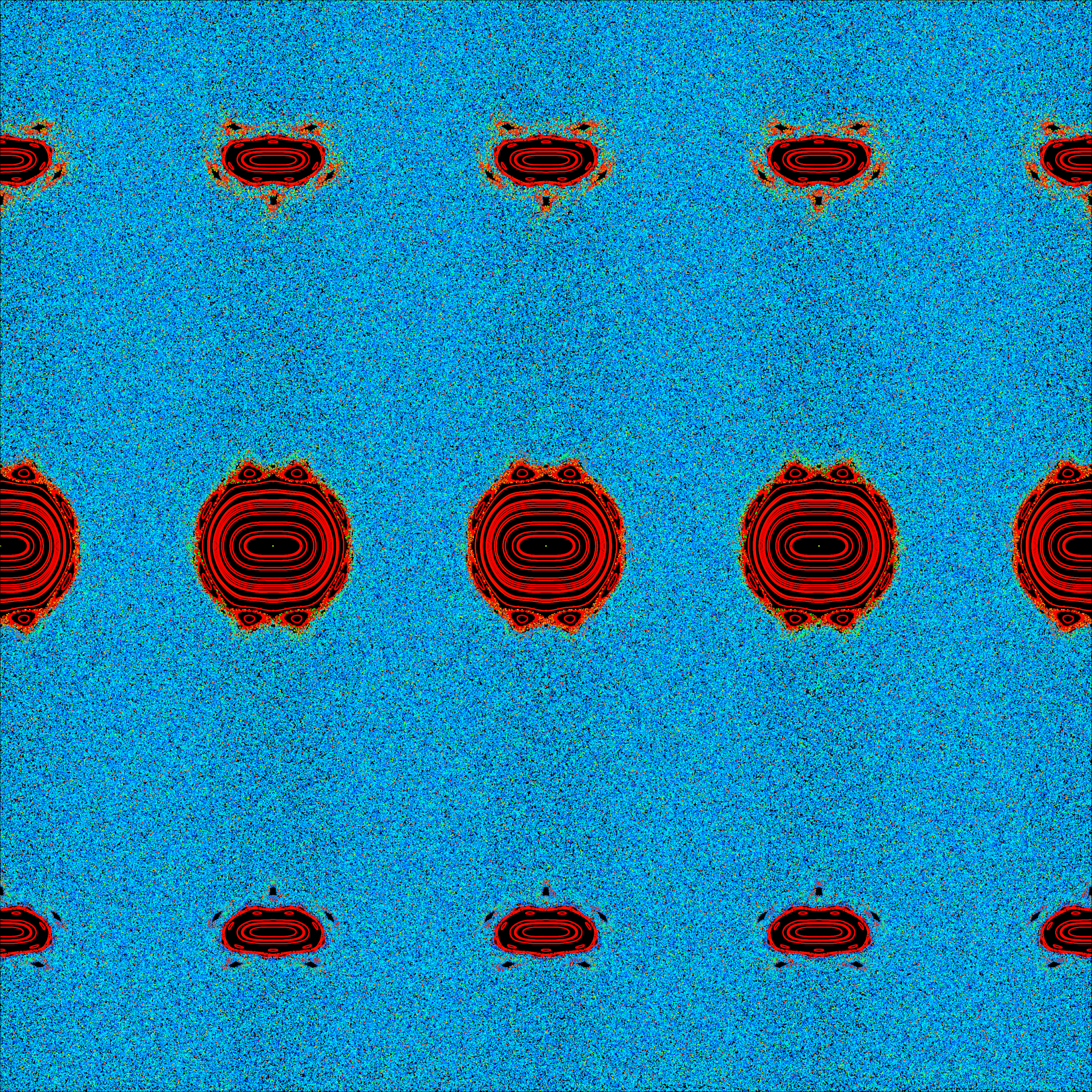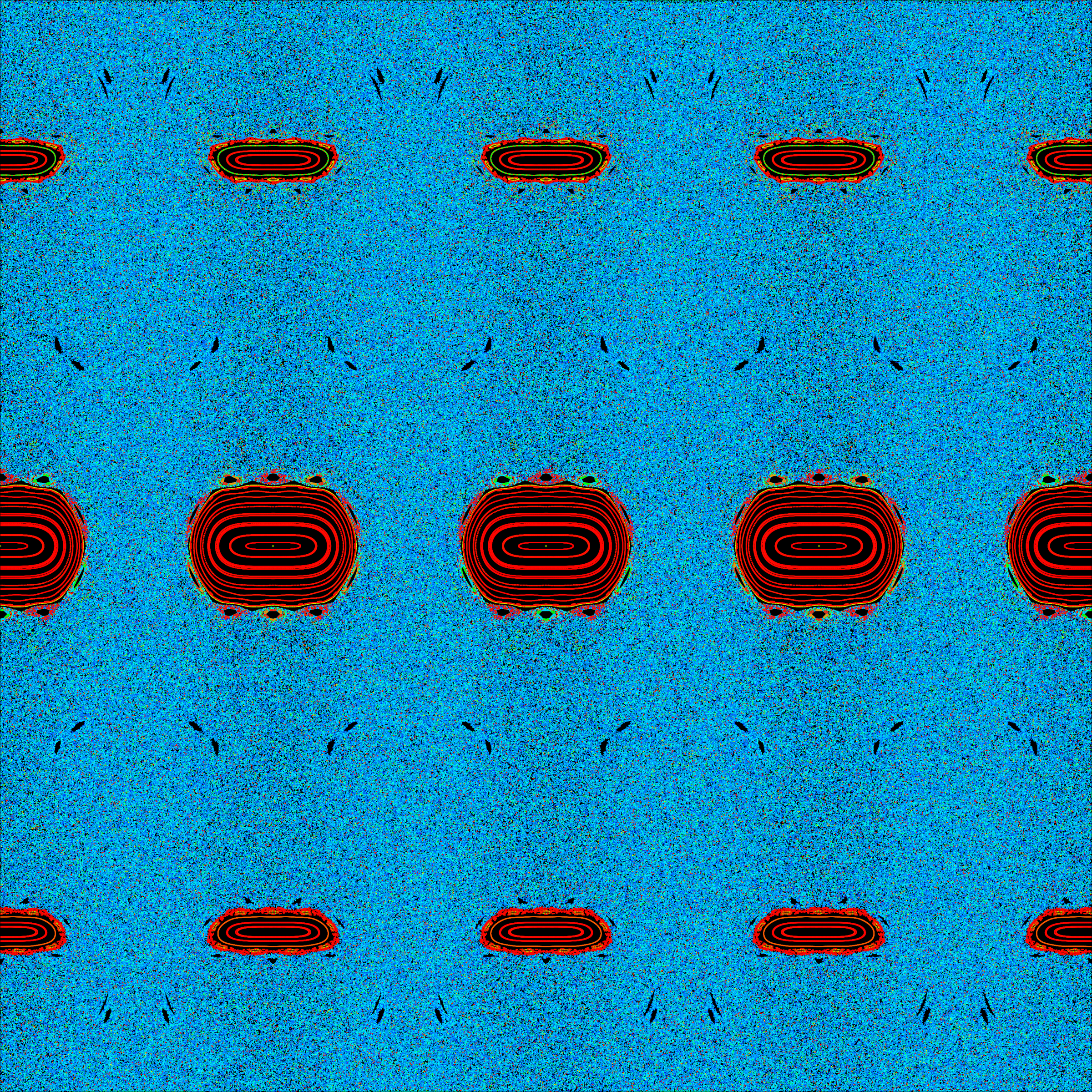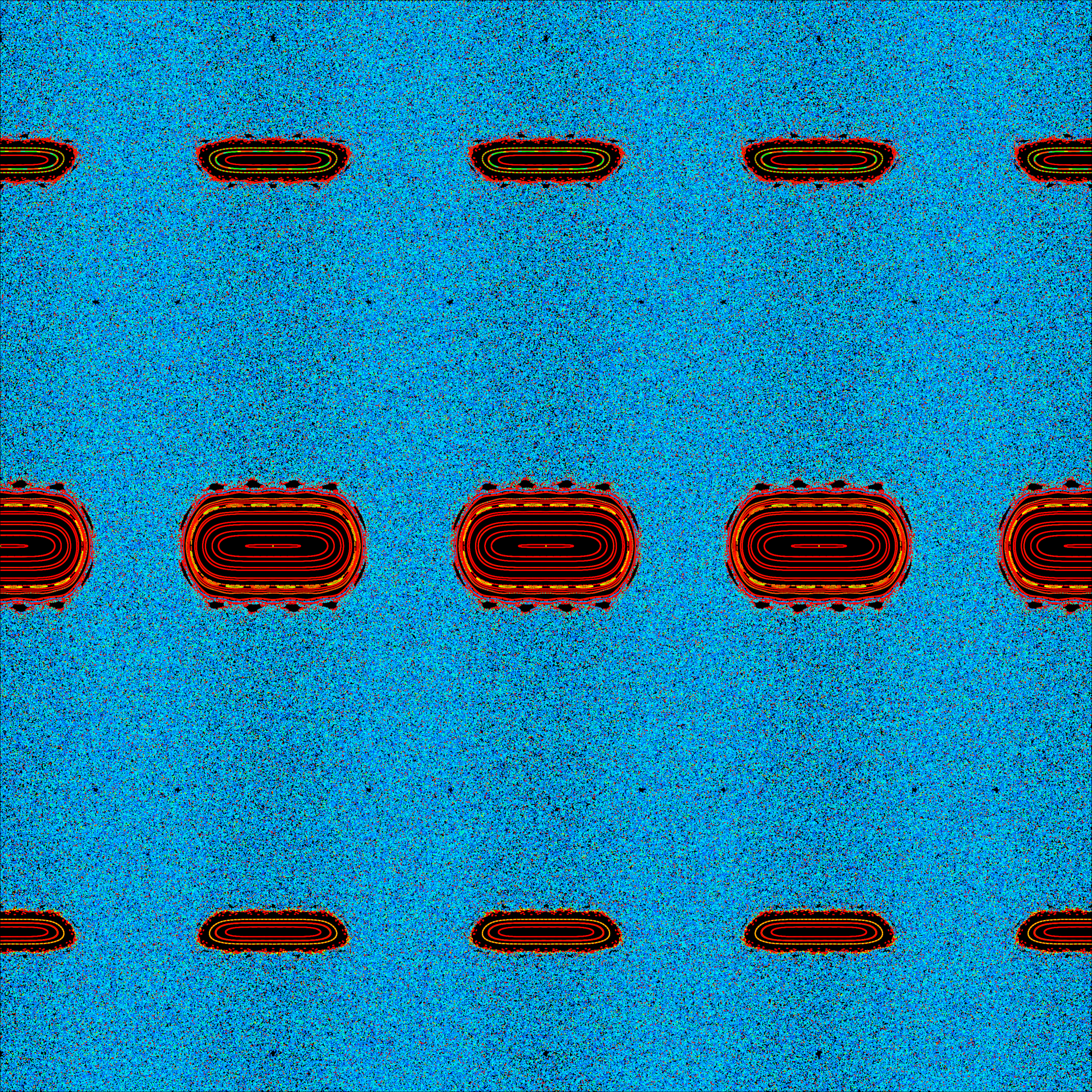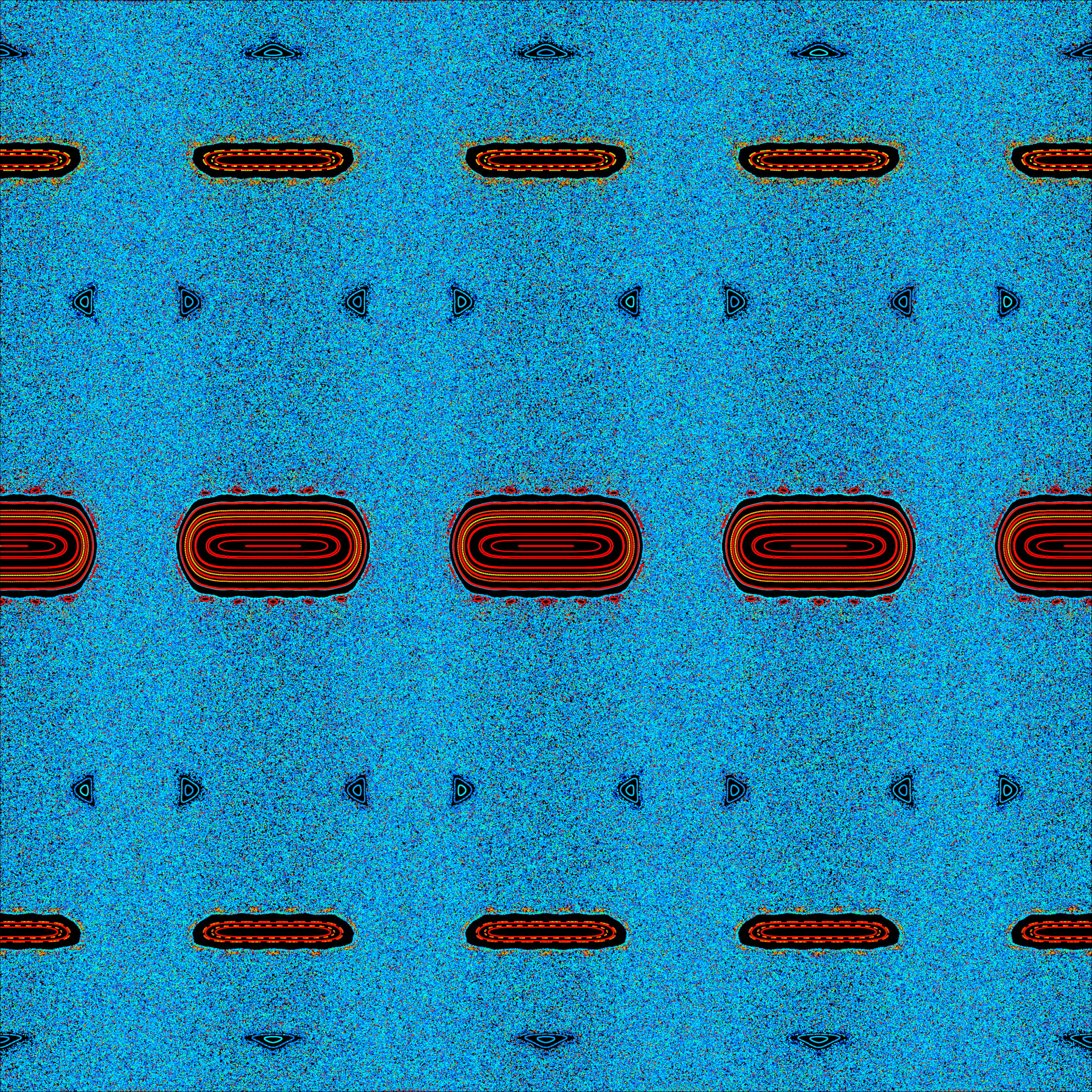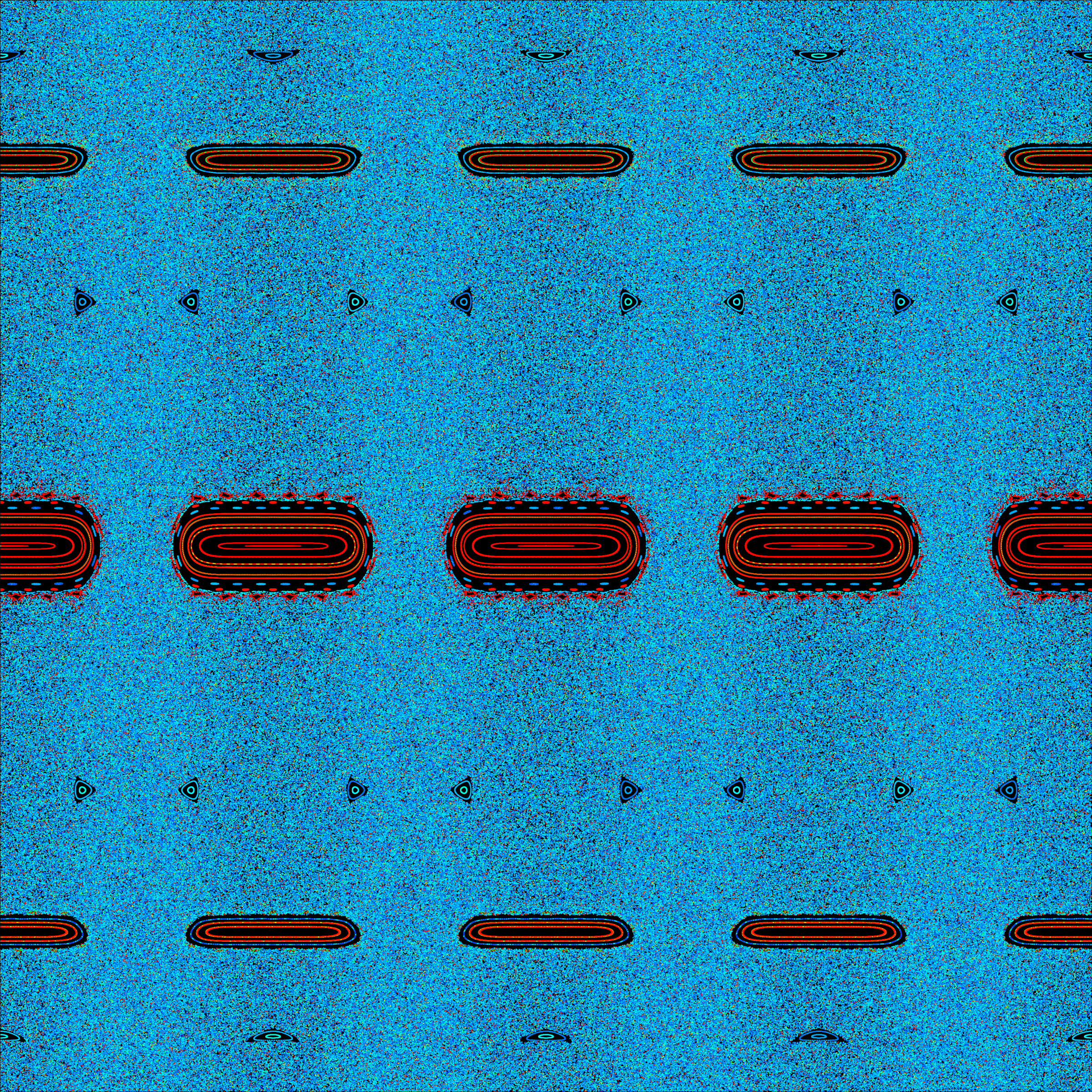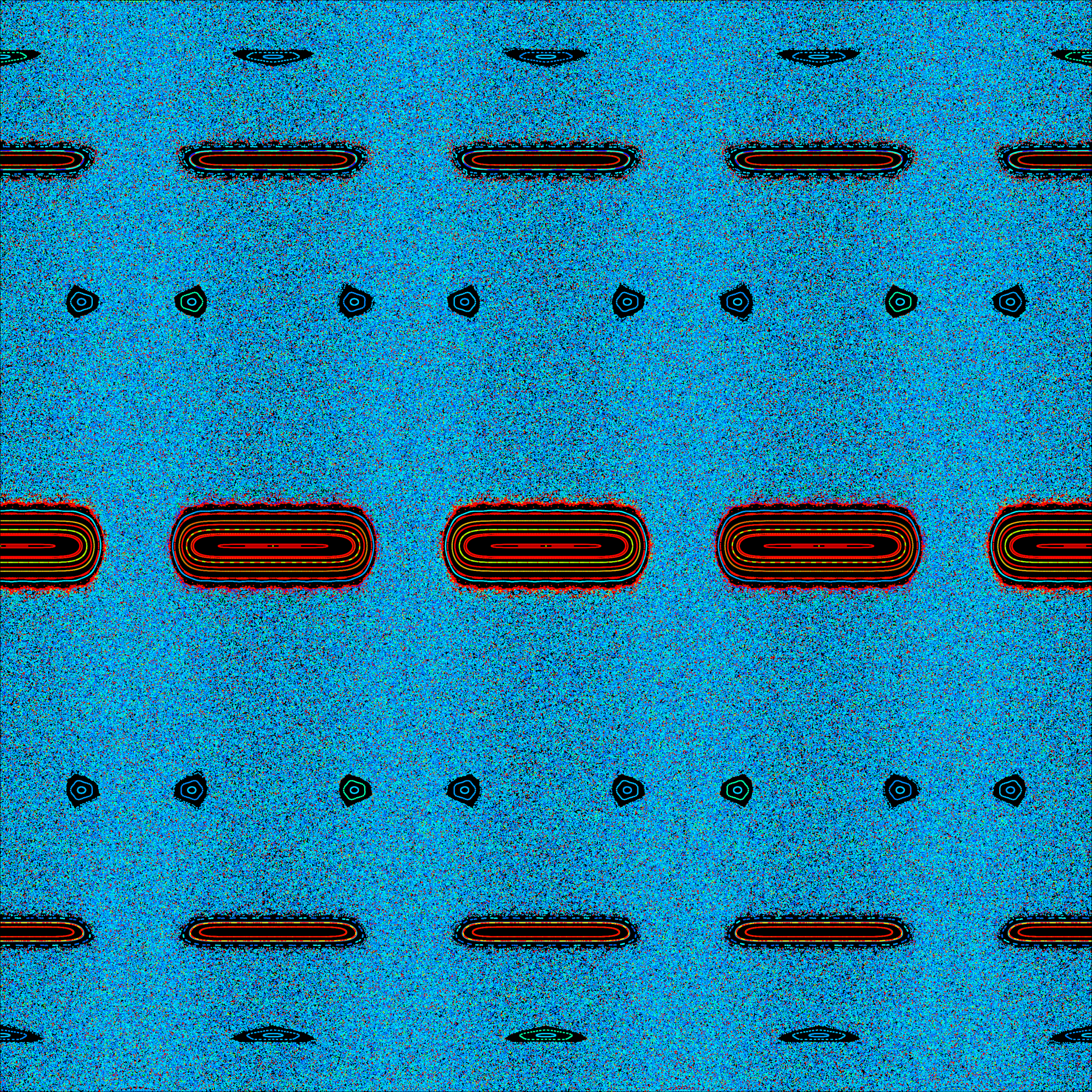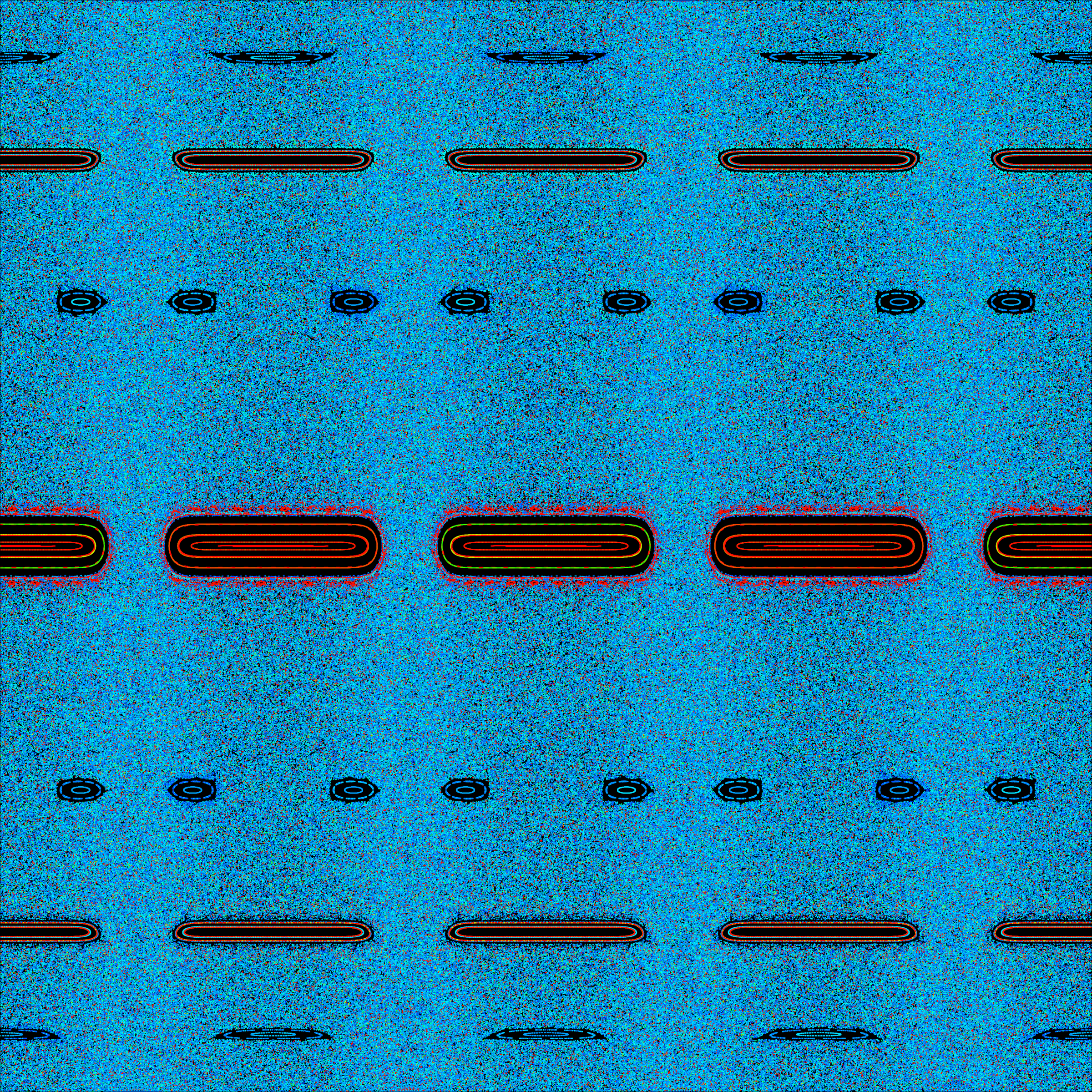Posted April 20, 2021